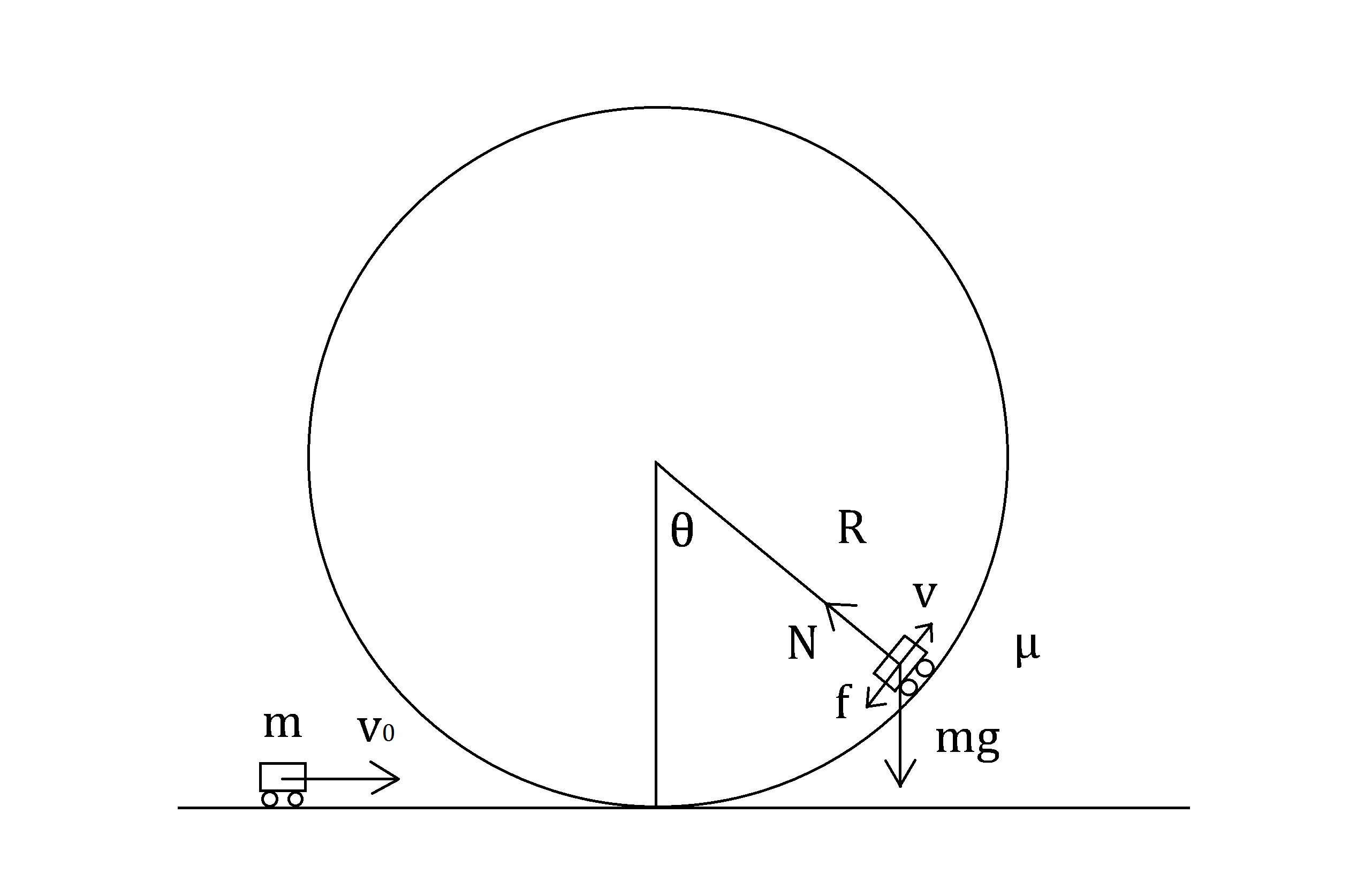# Circular Roller Coaster

Back

SIMIODE UPDATE

1-120-S-CircularRollerCoaster-StudentVersion

1-120-S-CircularRollerCoaster-StudentVersion

1-120-T-CircularRollerCoaster-TeacherVersion

1-120-T-CircularRollerCoaster-TeacherVersion

Circular Roller Coaster

Article published in Centennial High School Math Journal. Link to journal webpage.

Objective: Analyze an interesting variation to a standard textbook problem.

Mathematical Concepts:

1. Fundamental theorem of calculus
2. First-order linear differential equation
3. Integrating factor
4. Integration by parts

Consider a cart of mass$m$ moving through a vertical circle of radius$R$. We will assume the size of the cart is much smaller than the circle and neglect the rotational inertia of the cart’s wheels. The acceleration due to gravity is$g$.A typical textbook problem is to calculate the minimum initial velocity of the cart for it to stay on the track throughout the loop. The critical point is analyze is when the cart is at the top of the track. In that case, the normal force can only point downwards.Suppose the cart has initial velocity$v_0$. By conservation of energy:$\frac{1}{2}m{v_0}^2=mg(2R)+\frac{1}{2}mv^2$

For circular motion at the top of track:$mg+N=\frac{mv^2}{R}$

The key realization is that$N \geq 0$ and the minimum velocity will cause the cart to be just in contact with the track, that is$N=0$.$N=0$:$mg=\frac{mv^2}{R}$$v=\sqrt{gR}$

This is velocity of the cart at the top of the circle.$\frac{1}{2}m{v_0}^2=2mgR+\frac{1}{2}mgR=\frac{5}{2}mgR$$v_0=\sqrt{5gR}$

To analyze the entire system of motion, we can describe the location of the cart with angle$\theta$:By conservation of energy:$\frac{1}{2}m{v_0}^2=mgR(1-\cos{\theta})+\frac{1}{2}m{v}^2$$v^2={v_0}^2-2gR(1-\cos{\theta})$

Equation of motion:$v=R\dot{\theta}$$\omega_0 \equiv \frac{v_0}{R}$$\dot{\theta}=\sqrt{{\omega_0}^2-\frac{ 2g(1-\cos{\theta})}{R}}$

Normal force:$N-mg\cos{\theta}=\frac{mv^2}{R}$$N-mg\cos{\theta}=\frac{m{v_0}^2}{R}-2mg(1-\cos{\theta})$$N=\frac{m{v_0}^2}{R}+(3\cos{\theta}-2)mg$

Centripetal acceleration:$a_c=\frac{v^2}{R}=\frac{{v_0}^2}{R}-2g(1-\cos\theta)$

Tangential acceleration:$a_t=\frac{-mg\sin{\theta}}{m}=-g\sin{\theta}$

Now consider a more interesting track with friction present. The coefficient of friction is$\mu$ between the cart and the circular track. Let’s analyze this system as we did before.By conservation of energy:$\frac{1}{2}m{v_0}^2=mgR(1-\cos{\theta})+\frac{1}{2}m{v}^2+W_f$$W_f$ is the energy dissipated by friction which is given by:$W_f=\int_{0}^{\theta}\mu NR\,d\theta'$

Friction only affects the tangential acceleration so the centripetal acceleration stays the same:$N-mg\cos{\theta}=\frac{mv^2}{R}$

Substituting back$W_f$ and differentiating once with respect to$\theta$:$0=mgR\sin{\theta}+mv\frac{dv}{d\theta}+\mu N R$$0=mgR\sin{\theta}+mv\frac{dv}{d\theta}+\mu \left(mg\cos{\theta}+\frac{mv^2}{R}\right) R$$0=gR(\sin{\theta}+\mu\cos{\theta})+v\frac{dv}{d\theta}+\mu v^2$

A helpful substitution$u=v^2$:$u'=2vv'$$0=gR(\sin{\theta}+\mu\cos{\theta})+\frac{u'}{2}+\mu u$

This first-order linear differential equation is not separable, but we can make use of an integrating factor.$y'+p(t)y=g(t)$$\mu(t)=e^{\int p(t)\,dt}$

To avoid confusion, let’s use$\delta(\theta)$ as our integrating factor.$u'+2\mu u=-2gR(\sin{\theta}+\mu\cos{\theta})$$\delta(\theta)=e^{\int 2\mu d\theta}=e^{2\mu\theta}$$\frac{d}{d\theta}\left(e^{2\mu\theta}u\right)=-2gRe^{2\mu\theta}(\sin{\theta}+\mu\cos{\theta})$$e^{2\mu\theta}u=-2gR\int e^{2\mu\theta}(\sin{\theta}+\mu\cos{\theta})\,d\theta$

Integrating by parts:$\int e^{2\mu\theta}\sin{\theta}\,d\theta=\frac{e^{2\mu\theta}}{2\mu}\sin{\theta}-\frac{1}{2\mu}\int e^{2\mu\theta}\cos{\theta}\,d\theta$$\int e^{2\mu\theta}\cos{\theta}\,d\theta=\frac{e^{2\mu\theta}}{2\mu}\cos{\theta}+\frac{1}{2\mu}\int e^{2\mu\theta}\sin{\theta}\,d\theta$$\left(1+\frac{1}{4\mu^2}\right)\int e^{2\mu\theta}\sin{\theta}\,d\theta=\frac{e^{2\mu\theta}}{2\mu}\left(\sin{\theta}-\frac{\cos{\theta}}{2\mu}\right)$$\left(1+\frac{1}{4\mu^2}\right)\int e^{2\mu\theta}\cos{\theta}\,d\theta=\frac{e^{2\mu\theta}}{2\mu}\left(\cos{\theta}+\frac{\sin{\theta}}{2\mu}\right)$$\int e^{2\mu\theta}\sin{\theta}\,d\theta=\frac{e^{2\mu\theta}(2\mu\sin{\theta}-\cos{\theta})}{1+4\mu^2}$$\int e^{2\mu\theta}\cos{\theta}\,d\theta=\frac{e^{2\mu\theta}(2\mu\cos{\theta}+\sin{\theta})}{1+4\mu^2}$

Substitute results:$e^{2\mu\theta}u=-2gR\left(\int e^{2\mu\theta}\sin{\theta}\,d\theta+\mu\int e^{2\mu\theta}\cos{\theta}\,d\theta\right)$$e^{2\mu\theta}u=-2gR\left[\frac{e^{2\mu\theta}(2\mu\sin{\theta}-\cos{\theta})}{1+4\mu^2}+\frac{\mu e^{2\mu\theta}(2\mu\cos{\theta}+\sin{\theta})}{1+4\mu^2}\right]+C$$e^{2\mu\theta}u=-2gR\left[\frac{e^{2\mu\theta}(3\mu\sin{\theta}-\cos{\theta}+2\mu^2\cos{\theta})}{1+4\mu^2}\right]+C$$u=-2gR\left[\frac{(3\mu\sin{\theta}-\cos{\theta}+2\mu^2\cos{\theta})}{1+4\mu^2}\right]+Ce^{-2\mu\theta}$$u=Ce^{-2\mu\theta}+\frac{2gR}{1+4\mu^2}[(1-2\mu^2)\cos{\theta}-3\mu\sin{\theta}]$$v^2=Ce^{-2\mu\theta}+\frac{2gR}{1+4\mu^2}[(1-2\mu^2)\cos{\theta}-3\mu\sin{\theta}]$

Initial condition:$v=v_0$ at$\theta=0$${v_0}^2=C+\frac{2gR}{1+4\mu^2}(1-2\mu^2)$$C={v_0}^2-\frac{2gR(1-2\mu^2)}{1+4\mu^2}$$v^2=\left[{v_0}^2-\frac{2gR(1-2\mu^2)}{1+4\mu^2}\right]e^{-2\mu\theta}+\frac{2gR}{1+4\mu^2}[(1-2\mu^2)\cos{\theta}-3\mu\sin{\theta}]$

Equation of Motion:$R^2\dot{\theta}^2=\left[{v_0}^2-\frac{2gR(1-2\mu^2)}{1+4\mu^2}\right]e^{-2\mu\theta}+\frac{2gR}{1+4\mu^2}[(1-2\mu^2)\cos{\theta}-3\mu\sin{\theta}]$

Normal force:$N=\frac{mv^2}{R}+mg\cos{\theta}$

Centripetal acceleration:$a_c=\frac{v^2}{R}$

Tangential acceleration:$a_t=-\frac{mg\sin{\theta}+\mu N}{m}=-g(\sin{\theta}+\mu\cos{\theta})-\frac{\mu v^2}{R}$

Verify our derivation is correct by looking at$\mu = 0$. It should reduce to the frictionless case.$v^2={v_0}^2-2gR+2gR\cos{\theta}$